United States Department of LaborThe .gov means it's official.
Federal government websites often end in .gov or .mil. Before sharing sensitive information, make sure you're on a federal government site.The site is secure.
The https:// ensures that you are connecting to the official website and that any information you provide is encrypted and transmitted securely.

## Modeled Wage Estimates: Calculation

To calculate the Modeled Wage Estimates (MWE) the occupational and geographic detail from the Occupational Employment Wage Statistics (OEWS) program are combined with information on job characteristics from the National Compensation Survey (NCS). The Handbook of Methods for the OEWS and the NCS provide information regarding each survey’s calculation. The calculation details for the estimates and relative standard errors are covered in this section.

### Combining OEWS and NCS data

The method for calculating wage estimates by job characteristic generally follows the procedures and formula that the OEWS program uses to calculate its estimates (see Calculation) for the mean hourly wage, with an additional step to incorporate the information about job characteristics from the NCS. In the OEWS, wage rates of workers are reported as either grouped data across 12 consecutive, nonoverlapping wage intervals or as individual wage rates. The additional step fully allocates the OEWS employment counts across the possible values for each job characteristic and wage interval. This ensures wage estimates by job characteristic will be consistent with the OEWS data by area, occupation, and wage interval, thereby taking full advantage of the OEWS sample size (approximately 1.2 million establishments).

Table 1. OEWS wage intervals, November 2019–May 2020 panels
Interval Wages
Hourly wage Annual wage

Range A

Under $9.25 Under$19,240

Range B

9.25 – 11.99 19,240 – 24,959

Range C

12.00 – 15.49 24,960 – 32,239

Range D

15.50 – 19.74 32,240 – 41,079

Range E

19.75 – 25.49 41,080 – 53,039

Range F

25.50 – 32.74 53,040 – 68,119

Range G

32.75 – 41.99 68,120 – 87,359

Range H

42.00 – 53.99 87,360 – 112,319

Range I

54.00 – 69.49 112,320 – 144,559

Range J

69.50 – 89.49 144,560 – 186,159

Range K

89.50 – 114.99 186,160 – 239,199

Range L

115.00 and over 239,200 and over

Source: U.S. Bureau of Labor Statistics, Occupational Employment and Wage Statistics.

The method also takes advantage of the NCS information about the relationship between the job characteristic and the wage rate, a relationship that is reflected by the differences in the NCS proportions for the job characteristics across the OEWS wage intervals. For example, if there is a tendency for wage rates to be lower for part-time workers than for full-time workers in the NCS data for the occupation, the proportion of part-time workers will tend to be higher for the lower wage intervals and lower for the higher wage intervals. This difference will translate into a lower estimate for the mean hourly wage rate for part-time workers than for full-time workers.

The MWE requires assumptions related to the calculation of the proportion for the characteristics, primarily to deal with the much smaller responding sample size, approximately 14,000 responding establishments, for the NCS relative to the OEWS survey. An establishment’s employment count for an occupation in a wage interval is allocated based on the proportion for the job characteristic among NCS observations from the same area and occupation and with a wage rate within the wage interval. Thus, the estimation method assumes that an occupation’s proportion for the job characteristics apply uniformly to all establishments within the area. When matching OEWS establishments to the NCS proportions, the occupation is defined by the detailed (six-digit) Standard Occupational Classification (SOC) code, and area is defined as 1 of the 24 NCS sample areas. These 24 areas comprise the 15 largest metropolitan areas plus the balance of the 9 Census divisions, where the balance of a Census division includes all areas in the division except the 15 largest areas.

### Mean hourly wage estimation formula

The estimation domains for the MWE are occupational domains within geographic domains. Most occupational domains are two-- or six-digit occupational codes as defined by the Standard Occupational Classification (SOC) system. The rest are aggregations of various detailed (six-digit) occupations as defined by the OEWS program⁠. The geographic domains include 606 metropolitan and nonmetropolitan areas, 50 states and Washington D.C., and the nation. The areas include metropolitan areas and balance of state areas (referred to as nonmetropolitan areas herein). The MWE are computed for the following job characteristics: bargaining status (union and nonunion), work status (full- and part-time), basis of pay (time and incentive), and work levels (1 through 15, and not able to be leveled) as seen in exhibit 1. Estimates are also computed for full-time and part-time by work level. This yields 54 job characteristics for each estimation domain.

Exhibit 1. Components of job characteristics
Job characteristic Components Number of characteristics

Bargaining status

Union and nonunion 2

Work status

Full- and part-time 2

Basis of pay

Time and incentive 2

Work level

Levels 1 through 15, and not able to be leveled 16

Work level, full-time only

Levels 1 through 15, and not able to be leveled 16

Work level, part-time only

Levels 1 through 15, and not able to be leveled 16

Source: U.S. Bureau of Labor Statistics.

The MWE mean hourly wage for a domain D and job characteristic C is given by: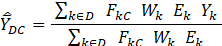For OEWS interval data:

k        is an OEWS establishment occupation wage interval

Yk       is the NCS interval-mean wage that is associated with k

For OEWS point data:

k        is an OEWS individual wage record in an OEWS establishment occupation

Yk       is the OEWS individual wage rate for k

For the other variables:

D        is an occupational group in a geographic area

C        is a job characteristic

FkC     is the MWE characteristic proportion for C associated with k

Wk      is the OEWS weight for the OEWS establishment containing k

Ek       is the OEWS employment of k

The characteristic proportion F for k and C is computed from the NCS data: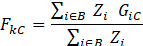where

i          is an individual wage record in an NCS sampled job (quote)

B         is the characteristic-proportion cell associated with k and C is the NCS individual weight for i

The characteristic-proportion cells B are initially broken out by OEWS panel, wage interval, six-digit SOC code, and NCS sample area as seen in exhibit 2. Yet if there are fewer than three NCS sampled jobs (quotes) in the cell, it is collapsed, and the characteristic proportion is recomputed using data from the collapsed cell. If necessary, NCS sample areas are first collapsed into Census divisions, then into Census regions, and then to the nation. If needed, the six-digit SOCs are then collapsed into major occupational groups (two-digit SOC). Finally, the major occupational groups are collapsed. If the collapse pattern is exhausted, the characteristic proportions are set to zero.

GiC       is 1 if the NCS sampled job (quote) containing i has worker characteristic C; otherwise let GiC = 0.

Zi        is the NCS individual weight for i

Exhibit 2. Wage interval per characteristics
Collapse hierarchy

1

Wage interval, six-digit SOC occupation, detailed area (one of the 24 areas)

2

Wage interval, six-digit SOC occupation, census geographical division

3

Wage interval, six-digit SOC occupation, census geographical region

4

Wage interval, six-digit SOC occupation

5

Wage interval, major occupation group

6

Wage interval

Source: U.S. Bureau of Labor Statistics.

If k is an establishment occupation wage interval, the NCS samples are used to obtain the interval mean wage. Next, an initial mean wage is computed for each OEWS panel and wage interval using this formula: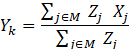where

j         =    individual wage record in an NCS sampled job (quote)

M       =    interval-mean cell, associated with k and C

Zj       =    NCS individual weight for j

Xj       =    NCS mean hourly wage for j

The interval means Yk for the five older OEWS panels are then aged forward using the Employment Cost Index (ECI).

Wages below the federal minimum wage are adjusted up to the federal minimum wage and included in interval 1. In some instances, state minimum wages exceed the lower bound of interval 1 or even interval 2. In these situations, the interval mean wages are shifted up to reflect the minimum wage. The interval mean wage may also be replaced with the weighted average wage, weighted by employment. It is calculated as the total of hourly wages for an occupation with that characteristic divided by the total employments for an occupation with that characteristic.

### Pooling

To increase the number of publishable estimates several NCS samples are combined with the full OEWS sample when calculating the MWE. Combining several sample groups—pooling—involves updating and using previously collected data from inactive survey establishments in conjunction with data from establishments in current (active) collection to produce estimates for a particular reference period. The 2014 and 2015 reference period estimates were limited to using data from establishments in active collection. However, starting with the 2016 reference period, pooling was implemented, which nearly doubled the number of published estimates.

NCS samples are considered active if they contribute to the Employment Cost Index (ECI). Every year, one group of establishments are sampled, initiated, and rotated into the active sample used to calculate the December reference period of the ECI. It remains active for 3 years until it is rotated out and then considered inactive.

### Aging

To use the inactive sampled groups, aging factors are calculated and applied to the hourly wages to produce estimates for a particular reference period. That is, the previous sampled hourly wages are adjusted using the current period ECI. Because of the differences in reference period, part of the active sample may also require de-aging to reflect the hourly wages of a prior reference period. The aging factors are calculated using the ECI for each of the nine published occupational groups. The ECI index values are used from the current reference period (panel p) as well as for the 2 prior years (panel p-1 and panel p-2) and for the future year (panel p+1). The aging (and de-aging) factors are obtained by dividing the ECI panel p values (of the current reference period) by the ECI panel p-1 values, the ECI panel p-2 values and the ECI (panel p+1) values, that correspond to the same occupational group.

For example, the ECI estimate for the management, business, and financial occupational group for the reference period June 2016 (panel p) is 126.8, and for the reference period September 2015 (panel p-1) is 125.2. Therefore, the aging factor is 126.8/125.2 = 1.013 The hourly wage rate of $35.00 for this occupational group would be increased by this aging factor such that the hourly wage rate for the reference period would be 1.013 x$35 = $35.45. ### Percent relative standard errors The variance estimate is a measure of mean squared deviation. However, the variance estimate is not directly comparable to the mean wage estimate because the mean wage is measured in dollars, whereas the mean squared deviation is measured in dollars squared. By taking the square root of the variance, its value becomes comparable to the mean wage estimate. The square root of the variance is the standard error. The standard error often varies across domains because of the size of the mean wage and not because of reliability issues. The standard error (SE) can be represented instead as a percentage of the mean wage. This approach allows better comparisons across domains and is referred to as the percent relative standard error (%RSE) or simply the relative standard error (RSE). To gauge the reliability of the MWE, RSEs are estimated and published. For example, if the average hourly wage for a MWE domain is$20.00 per hour, and its SE is \$0.20, then the RSE is 1.00 percent.

The SE can also be used to generate an estimated confidence interval as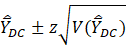where z depends on the desired confidence level.

For the 90-percent confidence level, z is about 1.645. To understand the estimated confidence interval, consider the following situation. Suppose the confidence level is 90 percent, and all samples can be selected, and their confidence intervals could be computed. Also, suppose these estimates are normally distributed. Then, the expectation is that 90 percent of these confidence intervals will contain the true population value. Estimates usually are not normally distributed, but for large sample sizes, the normal distribution is a good approximation of the true distribution. The estimate is more reliable when the variance and confidence are smaller.

The formula for the variance is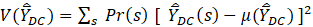where

D            is the MWE domain (occupational group in a geographic area),

C            is the job characteristic,

s             is a single sample,

Pr(s)       is the probability of selecting s,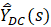is the mean wage estimator for the MWE program for domain (D) and characteristic (C) for a given sample (s), and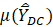is the expected value of the estimator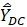,

where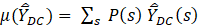.

The variance measures show how dispersed, on average, the sample estimates,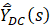, are from the population mean, m. When the variance is small, it means the sample estimates are tightly clustered and the mean wage estimator is therefore reliable. Conversely, when the variance is large, the sample estimates are more dispersed, and the estimator is less reliable. In other words, if a sample is chosen at random from the distribution, there is a higher chance of it being closer to m when the variance is low than when it is high.

For example, suppose there are 5 possible samples with estimates 1, 3, 5, 7, 9. The average of the estimates is 5, the variance is defined as the sum of each sample estimate’s distance from the mean squared divided by the average of the estimates which is  8 = 1/5 (4⁠2 + 2⁠2 + 0⁠2 + 2⁠2 + 4⁠2). The SE is calculated from the square of the variance which is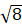≈ 2.8. The RSE is the SE divided by the average of the estimates, which is 2.8/5 = 56 percent. Now, suppose the estimates were instead 2, 4, 5, 6, 8. In this case, the average is 5, the variance is 4, such that the SE is 2, and the RSE is 40 percent. It is still possible to choose an outlier sample estimate (for example, 8 or 9), but the chances of being close to the mean (5) is greater in the second case, the one with lower variance.

The variance is based on the distribution of estimates across all possible samples. Because there is only one sample in practice, the variance cannot be calculated directly; instead, it is estimated. The variance estimator used for the MWE is called Fay’s method of balanced repeated replication (Fay’s BRR). It is like a calculated variance, except the sampling distribution used in the variance formula is replaced with a proxy distribution, whose elements are called replicates. Also, the value m is replaced with the estimate (also called the full sample estimate). While the variance is a function of the distribution of sample estimates, the Fay’s BRR variance estimator is a function of the distribution of replicate estimates.

The Fay’s BRR variance estimate is 4 times the average, over all replicates, multiplied by the squared deviation of the replicate estimates from the full sample estimate. The factor of 4 is used to properly scale the result. Each replicate estimate is found by perturbing the sampling weights and then recomputing the MWE estimate. Half the observations have their weights increased by 50 percent, whereas the remainder have their weights decreased by 50 percent.

The formula for the Fay’s BRR variance estimator is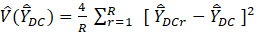where

r          is a single replicate,

R         is the number of replicates,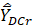is the replicate estimate for domain D and job characteristic C, and replicate r, andis the full sample estimate for domain D and job characteristic C.

The values above all apply to a single sample s, but this symbol is dropped for simplicity.

For the full sample estimate, the sampling weights are not perturbed. For the replicate estimates, the same formula as the full sample estimate is used, yet the sample weights are perturbed by +/- 50 percent. The weight perturbations of +/- 50 percent only capture about half of the deviation that would likely occur in a real sampling distribution. The standard error estimate must then be doubled to compensate, hence the factor of 4 is needed to properly scale the results.

The pattern of weight perturbation is designed to mimic the sampling variability that would occur if it were possible to draw a new sample, and it is done in a balanced fashion. To capture the structure of the sample design, variance strata are used to do the perturbations. Ideally, variance strata would mirror the sampling strata. Yet in practice, sampling strata usually need to be collapsed to reduce the total number of variance strata, H. In some cases, though, they are separated to increase the precision of the variance estimator.

Once variance strata are defined, each stratum is split into two variance primary sampling units (PSUs), labeled PSU-1 and PSU-2. For each replicate and variance stratum, a decision is made to upweight PSU-1 and downweight PSU-2, or either upweight PSU-2 and downweight PSU-1. If there are R replicates and H variance strata, these choices can be arranged in a matrix with R rows and H columns. Suppose the matrix value is 1 if PSU-1 was upweighted and downweighted otherwise. The perturbation pattern is said to be balanced if this matrix has orthogonal column vectors (i.e., the inner product of any two columns is zero). Note that the number of replicates R will always be greater than or equal to the number of strata H. So, the smaller H and R are, the quicker it takes to run variances. In many cases, variance strata were collapsed to reduce the size of H and R. Yet if H is too small, accuracy is lost.

For the MWE, the variance strata for each area and occupational domain are an amalgam of variance strata from the OEWS and NCS. OEWS used the Taylor series variance estimation method, while NCS uses Fay’s BRR.

When estimating the MWE for states, metropolitan, and nonmetropolitan areas, the corresponding NCS area stratum and PSU definition are used; otherwise, the NCS national stratum and PSU definition are used. This ensures there are sufficient variance strata to produce reliable variance estimates. If only national strata and PSUs were used, then for some MWE domains, there would be insufficient NCS variance strata.

For the OEWS units, the OEWS variance strata are set equal to the OEWS sampling strata. First, the strata are collapsed to ensure at least two establishments per stratum (one PSU-1 and one PSU-2). Since after collapsing the strata, the OEWS sample for a domain often have too many strata, so the strata are further collapsed. For large domains (e.g., particularly with national estimates and major occupation groups), the collapsing is extreme. In many cases, however, a domain had too few strata, so they were split (only if there were at least 3 establishments in the stratum).

### Publication criteria

To consider an estimate for publication, three conditions must be satisfied: there must be sufficient OEWS data to support a published estimate for the occupation within a given geographic area; there must be sufficient NCS data for the occupation, job characteristic, and area contributing to the wage estimate; and the average hourly wage estimate of each domain must fit broadly within expectations based on knowledge of compensation data. For example, average hourly wages for full-time workers are generally higher than for part-time workers. Additionally, the relationship between wage rates and job characteristics within geographical locations are assumed to be similar as those from broader geographic areas. The publication criteria ensures that estimates meet confidentiality requirements as well as BLS standards for reliability.

###### Notes

 The OEWS collects data based on the number of workers corresponding to the wage interval Occupational Employment Report or as individual wage rates.

 There were approximately 12,600 private industry and about 1,400 state and local government establishments from 7 samples contributing to the 2020 MWE.

 In 2019, OEWS used a hybrid approach to publish estimates for data collected using SOC 2010 and SOC 2018.

 Information on the geographic areas included in the OEWS are available in the Design section of the OEWS Handbook of Methods.

 Work levels span from levels 1 to 15. There are instances where leveling information is not available for selected jobs, these observations are grouped, and wage estimates are published as not able to be leveled. For more information on the leveling process, see the Guide to Evaluating Your Firm’s Jobs and Pay.

 The OEWS data are collected via two semiannual panels and estimates are produced from six panels collected over a 3-year period. The scope of the survey section of the technical note provides additional information on the use of collection panels.

 For example, if the ECI increased by 2 percent over the 12-month period, the interval means are increased by 2 percent to make the wages current for the reference period. The aging factors vary by panel and major occupational group. See wage aging process in the Calculation section of the Occupational Employment and Wage Statistics Handbook of Methods.

 With the implementation of the OEWS model-based estimation methodology (MB3), the OEWS reliability estimates are produced using the bootstrap replication technique as indicated in the variance estimation section of the Survey Methods and Reliability Statement for the MB3 Research Estimates of the Occupational Employment and Wage Statistics Survey. Information on the use for the Taylor series to calculate measures of reliability are available through the archived version of the Handbook of Methods.